# Tau versus Pi

Today, two of my friends independently sent me a story about Tau Day which I had hitherto never heard of. One of them asked for me comment about whether this had any point to it. At first I thought the article was just mathematical trolling, thought about it a bit more, thought there might be a real point to it, thought some more and concluded it seemed rather silly.

The argument is about whether the mathematical constant pi, would be better being replaced throughout mathematics with an alternative tau, which is just twice pi (in other words, replacing pi everywhere with a half of this tau). It’s suggested that formulae with tau will be more simple.

Basic Geometry

So this is all about the fact that pi was defined historically as the ratio of the circumference to the diameter of the circle, a very old classical reference stemming back to Greek geometry (incidentally pi is also known as Archimedes’ constant since he attempted to calculate an approximation to it). Once upon a time, the formula used in schools would have been: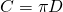related the circumference C to the diameter D. But generally now, we use the radius r rather than the diameter. And so that gives us (for circumference and area):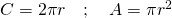The argument for tau begins by observing the extra 2 in the first formula, and wouldn’t be nicer if we just defined tau to be twice pi so that these formula would be so much nicer. Would they?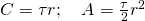Set aside for the moment the fact that pi is probably the most recognisable Greek letter in the world that speaks languages based on the Latin alphabet (aside from those that are, or appear to be the same). Set aside the fact that tau is used for other specific purposes in much of modern Mathematics, and in particular in the discipline of Topology. The first formula might be nicer, but the second one is probably worse, and by enough to make the improvement of the first rather parlous. OK. But the article talks about this being the problem behind radians, so maybe that’s where we get the big gain. Let’s explore that.

There’s nothing particularly clever about using degrees. It’s an arbitrary choice (360 degrees in a circle) that probably owes a lot to do with historical factors in one civilisation. It is true that when you start to do some significant mathematics with degrees, it starts to look quite unwieldy. The classic two formulae to consider are the length of an arc and area of a sector.

Suppose we have a circle of radius r and we want to work out the length of an arc (a part of the circumference) where the angle subtending this arc is theta degrees (don’t panic, no more Greek to come). Then in degrees the formula will be: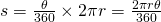The reason why is that the fraction on the left is the fraction of the relevant angle out of all the angle available, multiplied by the total arc length available (the whole circumference). The formula is not beautiful, and the similar formula for sector area is also a big ugly.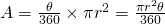You will note that in both cases there is a 360 on the bottom of the fraction and a 2 pi on the top. This looks like nature’s way of trying to tell us something. What would happen if we used an unit of angle so that, instead of having 360 of them in a circle, we had 2 pi of them in a circle (proponents of tau will just say tau of them in a circle)? The formula, derived using the same logic, become much nicer.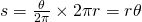So we get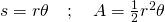Now these are beautiful, elegant formulae, and the underpinning of why radians (the unit of angle we are talking about here) are used instead of degrees in much of higher mathematics, the formula are much simpler (particularly true when using calculus). Also, look at that first formula, it has all the resonance of F = ma. 1 unit of arc length is found in a circle of radius 1 unit with an angle of 1 radian. So beautiful is this that it used as the definition of the radian in many books. So far, so good. Did we really need tau to produce these? Does it matter that it it tau and not pi that cancels out? I can’t see why.

Fourier Series

Another example owes to the work of Fourier, who showed that repeating patterns can be broken into sums of the most basic repeating functions, the ones that are most simple are the sine and cosine functions. These are used to model waves of any sort which are of course ubiquitous in nature. It turns out you can build up more odd shapes like triangular and square waves out of these sinusoidal ones. Being able to do this is important in many aspects of Science and Engineering, and eventually this theory leads to all sorts of cool stuff like the way data is compressed in photos and more.This graph is labelled in degrees, so you can imagine if we change it to radians as we should then the 360 would become 2 pi. This is where again, proponents of tau will argue that replacing the 360 with a simple tau makes things easier. And it does, if all you want to do is to label that diagram. But the foundation of Fourier theory is building functions up in combinations of these: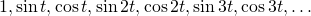The formulae you need to be able to deal with to do this are (among others):which I grant, strike fear into the hearts of many. But they don’t look nicer with tau (and are a little more awkward):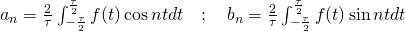Remember, the people arguing for tau are claiming it simplifies formulae, not making them look worse.

Euler’s Identity

Finally, I cannot leave this without talking about Euler’s identity considered by most mathematicians (including myself) to be one of the most beautiful results in Mathematics.This result can be written in a few ways, but this way is very commonly used. This is because in this form you can see how this identity connects the five most important numbers of Mathematics: 0, 1, pi, i and e. With tau, it just doesn’t have the same beauty: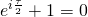so I will stick with pi. Thanks all the same.

Posted

in

by

Tags:

### 6 responses to “Tau versus Pi”

1.Clem Dickey

Euler’s identity, using tau, is “e to the i tau equals 1”. Or, if you must have a zero, “e to the i tau plus 0 equals 1”.

2.Colin Turner

That misses the point for me. The beauty isn’t just eliminating variables. It’s easy to produce more minimal relationships, it’s that the identity as is unifies elegantly the 5 most important numbers in mathematics.

3.Colin Turner

A bit laboured for me (adding a zero), but I take your point. I still don’t think it improves it.

4.soulcyon

The argument for Fourier is the only one that holds water.

5.Colin Turner

You might need to actually justify that comment to receive a meaningful reply…

6.Colin Turner

…guess what, I know area is an integral.

Anyway, what is your point? The relationship between 2 * pi * r and pi * r^2 and differentiation and integration is obvious.